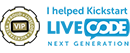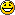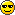## Image Manipulation

Visuals, audio, animation. Blended, not stirred. If LiveCode is part of your rich media production toolbox, this is the forum for you.

Moderators: Klaus, FourthWorld, heatherlaine, robinmiller, kevinmiller

bn
VIP Livecode Opensource BackerPosts: 3326
Joined: Sun Jan 07, 2007 9:12 pm
Location: Bochum, Germany

### Re: Image Manipulation

Hi,
I tried to speed bump the autobrighten handler

Code: Select all

``````function addFilter_AutoBrighten pImage,pStyle,pStyle2,tAmount
if pImage is empty then exit to top
put the imageData of pImage into tData
put the width of pImage into pWidth
put the height of pImage into pHeight

put pWidth * pHeight * 4 into tHowOften

repeat with tCounter = 2 to tHowOften step 4

put charToNum(char tCounter of tData) into tRed
put charToNum(char tCounter + 1 of tData) into tGreen
put charToNum(char tCounter + 2 of tData) into tBlue

-- -- see below for compounded formula
--  put (tRed + tGreen + tBlue)/3 into pHold
--  put pHold/255 into tPercent
--  put (tAmount*2) * tPercent into pHold
--  subtract tAmount from pHold

-- replaces above blocked code
put ((tAmount*2) * (((tRed + tGreen + tBlue)/3)/255))-tAmount into pHold

if pStyle is "1" then
-- Adjust High & Low Levels
-- put pHold into pHold -- not necessary
else if pStyle is "2" then
if (pHold < 0) then
put 0 into pHold
end if
else if pStyle is "3" then
if (pHold > 0) then
put 0 into pHold
end if
end if

put (16 + ((.183 * tRed) + (.614 * tGreen) + (.062 * tBlue))) into tY
put (128 + (((-.101) * tRed) + ((-.339) * tGreen) + (.439 * tBlue))) into tCb
put (128 + ((.439 * tRed) + ((-.339) * tGreen) + ((-.040) * tBlue))) into tCr

if pStyle2 is 1 then
else if pStyle2 is 2 then
subtract pHold from tY
end if

put numToChar(max(min(the round of ((1.164 * (tY - 16)) + (0.000 * (tCb - 128)) + (1.793 * (tCr - 128))),255),0)) into char tCounter of tData
put numToChar(max(min(the round of ((1.164 * (tY - 16)) + ((-0.213) * (tCb - 128)) + ((-0.533) * (tCr - 128))),255),0)) into char tCounter + 1 of tData
put numToChar(max(min(the round of ((1.164 * (tY - 16)) + (2.112 * (tCb - 128)) + (0.000 * (tCr - 128))),255),0)) into char tCounter + 2 of tData

end repeat

set the imageData of pImage to tData
return "Render Complete!"
this reduces the time for a 640 by 480 px image from 2500 milliseconds to 1600 milliseconds on my computer.
The speed increase comes at a cost: the code is hardly legible, would need commenting for maintenance. You can compare it to the current version of PhotoRoom. Just paste this code into the script of card main and block the current handler.

here is one version that takes out the conditionals of the inner repeat loop at the cost of being a lot longer, this one saves another 80 milliseconds on said dimensions.

Code: Select all

``````function addFilter_AutoBrighten pImage,pStyle,pStyle2,tAmount
if pImage is empty then exit to top
put the imageData of pImage into tData
put the width of pImage into pWidth
put the height of pImage into pHeight

if pStyle2 is 1 then
put 1 into tMultiply
else
put - 1 into tMultiply
end if

put pWidth * pHeight * 4 into tHowOften

if pStyle is 1 then
repeat with tCounter = 2 to tHowOften step 4

put charToNum(char tCounter of tData) into tRed
put charToNum(char tCounter + 1 of tData) into tGreen
put charToNum(char tCounter + 2 of tData) into tBlue

-- -- see below for compounded formula
--  put (tRed + tGreen + tBlue)/3 into pHold
--  put pHold/255 into tPercent
--  put (tAmount*2) * tPercent into pHold
--  subtract tAmount from pHold

-- replaces above blocked code
put ((tAmount*2) * (((tRed + tGreen + tBlue)/3)/255))-tAmount into pHold

--

put (16 + ((.183 * tRed) + (.614 * tGreen) + (.062 * tBlue))) into tY
put (128 + (((-.101) * tRed) + ((-.339) * tGreen) + (.439 * tBlue))) into tCb
put (128 + ((.439 * tRed) + ((-.339) * tGreen) + ((-.040) * tBlue))) into tCr

add (pHold * tMultiply) to tY

put numToChar(max(min(the round of ((1.164 * (tY - 16)) + (0.000 * (tCb - 128)) + (1.793 * (tCr - 128))),255),0)) into char tCounter of tData
put numToChar(max(min(the round of ((1.164 * (tY - 16)) + ((-0.213) * (tCb - 128)) + ((-0.533) * (tCr - 128))),255),0)) into char tCounter + 1 of tData
put numToChar(max(min(the round of ((1.164 * (tY - 16)) + (2.112 * (tCb - 128)) + (0.000 * (tCr - 128))),255),0)) into char tCounter + 2 of tData

end repeat
end if

if pStyle is 2 then
repeat with tCounter = 2 to tHowOften step 4

put charToNum(char tCounter of tData) into tRed
put charToNum(char tCounter + 1 of tData) into tGreen
put charToNum(char tCounter + 2 of tData) into tBlue

-- -- see below for compounded formula
--  put (tRed + tGreen + tBlue)/3 into pHold
--  put pHold/255 into tPercent
--  put (tAmount*2) * tPercent into pHold
--  subtract tAmount from pHold

-- replaces above blocked code
put ((tAmount*2) * (((tRed + tGreen + tBlue)/3)/255))-tAmount into pHold

put max(pHold,0) into pHold

put (16 + ((.183 * tRed) + (.614 * tGreen) + (.062 * tBlue))) into tY
put (128 + (((-.101) * tRed) + ((-.339) * tGreen) + (.439 * tBlue))) into tCb
put (128 + ((.439 * tRed) + ((-.339) * tGreen) + ((-.040) * tBlue))) into tCr

add (pHold * tMultiply) to tY

put numToChar(max(min(the round of ((1.164 * (tY - 16)) + (0.000 * (tCb - 128)) + (1.793 * (tCr - 128))),255),0)) into char tCounter of tData
put numToChar(max(min(the round of ((1.164 * (tY - 16)) + ((-0.213) * (tCb - 128)) + ((-0.533) * (tCr - 128))),255),0)) into char tCounter + 1 of tData
put numToChar(max(min(the round of ((1.164 * (tY - 16)) + (2.112 * (tCb - 128)) + (0.000 * (tCr - 128))),255),0)) into char tCounter + 2 of tData

end repeat
end if

if pStyle is 3 then
repeat with tCounter = 2 to tHowOften step 4

put charToNum(char tCounter of tData) into tRed
put charToNum(char tCounter + 1 of tData) into tGreen
put charToNum(char tCounter + 2 of tData) into tBlue

-- -- see below for compounded formula
--  put (tRed + tGreen + tBlue)/3 into pHold
--  put pHold/255 into tPercent
--  put (tAmount*2) * tPercent into pHold
--  subtract tAmount from pHold

-- replaces above blocked code
put ((tAmount*2) * (((tRed + tGreen + tBlue)/3)/255))-tAmount into pHold

--
put min(pHold,0) into pHold

put (16 + ((.183 * tRed) + (.614 * tGreen) + (.062 * tBlue))) into tY
put (128 + (((-.101) * tRed) + ((-.339) * tGreen) + (.439 * tBlue))) into tCb
put (128 + ((.439 * tRed) + ((-.339) * tGreen) + ((-.040) * tBlue))) into tCr

add (pHold * tMultiply) to tY

put numToChar(max(min(the round of ((1.164 * (tY - 16)) + (0.000 * (tCb - 128)) + (1.793 * (tCr - 128))),255),0)) into char tCounter of tData
put numToChar(max(min(the round of ((1.164 * (tY - 16)) + ((-0.213) * (tCb - 128)) + ((-0.533) * (tCr - 128))),255),0)) into char tCounter + 1 of tData
put numToChar(max(min(the round of ((1.164 * (tY - 16)) + (2.112 * (tCb - 128)) + (0.000 * (tCr - 128))),255),0)) into char tCounter + 2 of tData

end repeat
end if

set the imageData of pImage to tData
return "Render Complete!"
I don't think that it gets any faster, would be interested in a faster version though.
For each will probably not make it faster since Livecode is very fast at getting a char = byte. The reason I suppose is that it accesses individual bytes by some pointer arithmetic. For each is a lot faster for chunks greater than bytes e.g. words, lines, items because Livecode does a lot less counting compared to repeat with i = x to the number of words of y. Additionally you have to maintain your own counter to know what byte you are accessing since you don't want every byte sequentially. And if you want them sequentially you need triplets of chars for red, green and blue. (tried it but it gets a bit messy and is slower than above handlers.)

Timing is not accurate since it varies from run to run a bit, times in milliseconds are roundabout times but give a feeling for the relative speed of different handlers.

LIveCode is not fast enough to make image manipulation a real joy. But then consider the computation needed for an image 640 by 480 px. = 640*480 =307200 px =1228800 bytes. And you have to access them to read them, convert them with charToNum, do your computation and convert them back with numToChar and write them to the imageData. Unless Livecode provides a faster means of accessing imageData it is all we have.

Kind regards
Bernd

teacherguy
Posts: 377
Joined: Thu Dec 08, 2011 2:43 am

### Re: Image Manipulation

Thank you!!!teacherguy
Posts: 377
Joined: Thu Dec 08, 2011 2:43 am

### Re: Image Manipulation

bn if you have any inclination to speed bump

I wouldn't be unappreciative eitherdavid.silmanBUSrUeQ
Posts: 44
Joined: Thu Jul 19, 2012 1:49 pm

### Re: Image Manipulation

Hi Chris

This is an amazing program!
We're in the process of writing our own program and we need a decent image manipulation part, would we be able to include yours into our program?
If so, what do you want in return?

Many thanks
David

Klaus
Posts: 11438
Joined: Sat Apr 08, 2006 8:41 am
Location: Germany
Contact:

### Re: Image Manipulation

david.silmanBUSrUeQ wrote:If so, what do you want in return?
Don't think it will be more than your soul...cbodell
Posts: 61
Joined: Wed Nov 09, 2011 7:27 pm

### Re: Image Manipulation

Hi david.silmanBUSrUeQ

Feel free to use the code in anyway, no need for anything in return!

Chris Bodell

FourthWorld
VIP Livecode Opensource BackerPosts: 7188
Joined: Sat Apr 08, 2006 7:05 am
Location: Los Angeles
Contact:

### Re: Image Manipulation

@cbodell: Your work is wonderful and I'd like to move this thread into the new Community Projects section I just created. Does the stack now include an explicit license? As I recall, it seemed your intentions were that it be in the public domain, yes? If so, it would be very helpful if you could consider adding a note to that effect in the stack so others who get it can have clarity about the terms of use.
Community volunteer LiveCode Community Liaison

LiveCode development, training, and consulting services: Fourth World Systems: http://FourthWorld.com

capellan
Posts: 622
Joined: Wed Aug 15, 2007 11:09 pm
Contact:

### Re: Image Manipulation

Christopher Lee Bodell died on January 17, 2013
http://www.hughes-ransom.com/obituary/C ... /_/1314195

Klaus
Posts: 11438
Joined: Sat Apr 08, 2006 8:41 am
Location: Germany
Contact:

### Re: Image Manipulation

capellan wrote:Christopher Lee Bodell died on January 17, 2013
http://www.hughes-ransom.com/obituary/C ... /_/1314195
Oh boy, so young, so sad!Carpe diem, my friends, carpe diem (quam minimum credula postero)!